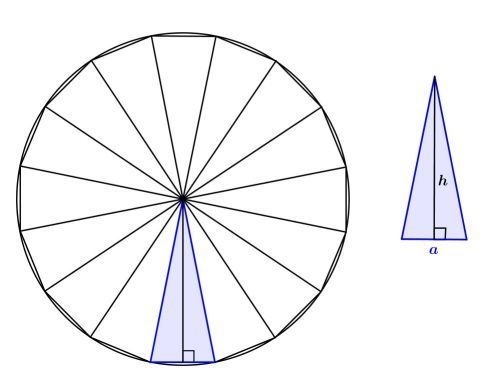# Explain please Area of circle

• Area of circle and stuff I am sooo confused about. Not really in any of the courses I've taken, but I've seen it and I'm confused.

Your confusion is normalYou can also imagine that a circle is a regular polygon with lots and lots of vertices (actually, infinitely many vertices). To find the area of a regular polygon, you cut it into isosceles triangles (see picture) and then sum up their areas. So the area of a polygon is equal to $$n\times(\frac{1}{2}ah)=\frac{h}{2}\times(na),$$ where $$n$$ - number of vertices, $$a$$ - side length and $$h$$ - distance from the center of polygon to its side. The term $$na$$ is the perimeter of the polygon. As the polygon becomes more and more like a circle, this value approaches the value of the circle's circumference, which is $$2\pi r,$$ and value of $$h$$ approaches the circle radius. So, substituting $$2\pi r$$ instead of $$na,$$ we get: $$\text{area of circle}=\frac{r}{2}\times(2\pi r)=\pi r^2.$$Studying this in more detail and discovering lots of new and very interesting things will be easier as you get older and study higher-level topics like functions, derivatives, integrals, and many other things.